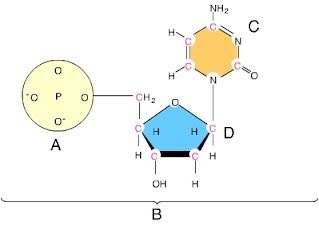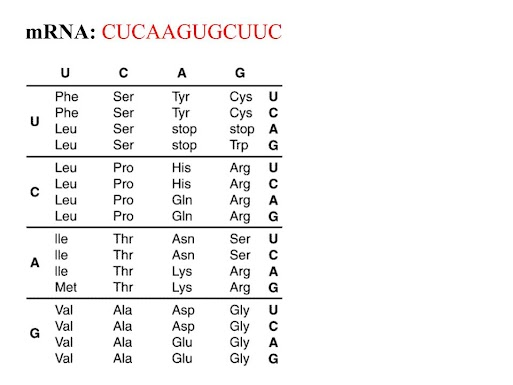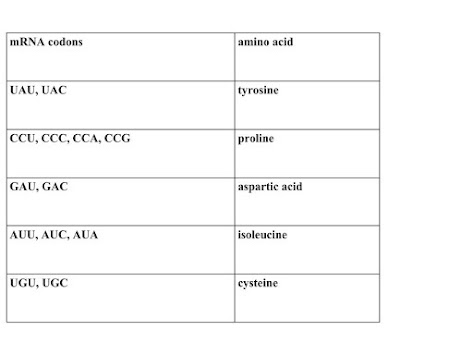DNA transcription/2017
Last Name *
First Name *
1. A generic RNA nucleotide is made up of the following parts. check all that apply.
2. RNA stands for
3. In a RNA strand, what base is NOT used?
4. What are the two steps in reading the DNA code? check all that apply
7. What are the 3 types of RNA? check all that apply
5.Check all that apply regarding RNA
6. If a DNA strand reading TTT GAG CCC ATA is transcribed, what mRNA would you make?
Diagram 28. If diagram 2 above were a RNA nucleotide, D is would be
9. If diagram 2 above were an RNA nucleotide, letter C could NOT be
10. . In a sample of DNA, 25% of the nitrogenous bases are thymine. That piece of DNA is transcribed. The resulting mRNA would be ____________
11. mRNA is made up of codons. How many bases in a codon?
12 What is produced during transcription?
13. Refer to the illustration below (Figure 1). What is the portion of the protein molecule coded for by the piece of mRNA given? You will need the decoder chart!
Figure 114. Refer to the illustration above (Figure 1). Which of the following would represent the strand of DNA from which the mRNA strand given was made?
15. Given the following mRNA: CUCAAGUGC. How many codons are present?
16. Refer to the illustration below (FIGURE 3). Suppose that you are given a protein containing the following sequence of amino acids: tyrosine, proline, aspartic acid, isoleucine, and cysteine. Use the portion of the genetic code given to determine which of the following contains a DNA sequence that codes for this amino acid sequence.
Figure 3